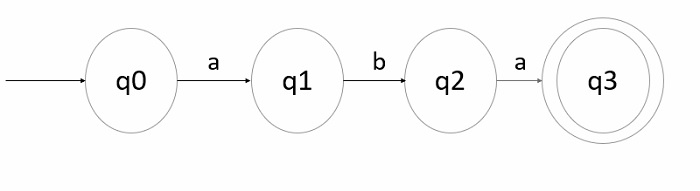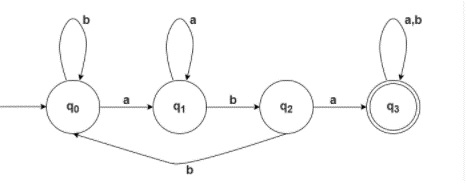# C program for DFA accepting all strings over w ‚àà(a,b)* containing ‚Äúaba‚Äù as a substring

## Problem

Design a DFA for the language L={w1abaw2 | w1,w2 –Ñ(a,b)*}, which means the DFA accepts all strings which contain “aba” as a substring.

## Solution

The strings that are accepted by language L= {aba,aabaa, aabab, babab, ababa, …….}

Step 1 − Transition diagram for minimal string (starting string) −If w1 and w2 are null then the string it generates is “aba” because w1, w2 ε(a,b)*

q0 is the initial state and q3 is the final state.

Step 2 − The final DFA for the given language is as follows −## Explanation

• qo is the initial state q0 on ‘a’ goes to q1 and on ‘b’ goes to q0, according to DFA each state has to generate a transition on both inputs.

• q1 on ‘a’ goes to q1 and on ‘b’ goes to q2, we have to keep in mind according to the given language we need to generate a substring “aba”.

• q2 on ‘a’ goes on q3 on ‘b’ goes to q0 state.

• q3 which is the final state, on input ‘a’ and ‘b’ goes to q3 only.

## Transition Table

Let’s see the transition table as follows −

Current stateInput aInput b
q0q1q0
q1q1q2
q2q3q0
*q3q3q3

## Example

Following is the C program for construction of DFA accepting all strings over w ε(a,b)* which contains “aba” as a substring -

Live Demo

#include <stdio.h>
#include <string.h>
void checkDFA(char s[] ) {
// storing initial state
int initialState = 0;
int previousState = initialState;
int finalState;
for(int i = 0; i < strlen(s); i++)    {
if((previousState == 0 && s[i] == 'a') || (previousState == 1 && s[i] == 'a')) {
finalState = 1;
}
if((previousState == 0 && s[i] == 'b') || (previousState == 2 && s[i] == 'b')) {
finalState = 0;
}
if(previousState == 1 && s[i] == 'b') {
finalState = 2;
}
if((previousState == 2 && s[i] == 'a') || (previousState == 3)) {
finalState = 3;
}
previousState = finalState;
}
if(finalState == 3) {
printf("given string is Accepted");
}
else
{
printf("given string is Not Accepted");
}
}
int main() {
// Given string
char s;
printf("implementation of DFA which having a sub string 'aba': enter a string:");
scanf("%s",s);
checkDFA(s);
return 0;
}

## Output

The output is as follows −

Run 1:
implementation of DFA which having a sub string 'aba':
enter a string:aba
given string is Accepted.
Run 2:
implementation of DFA which having a sub string 'aba':
enter a string:abba
given string is Not Accepted.